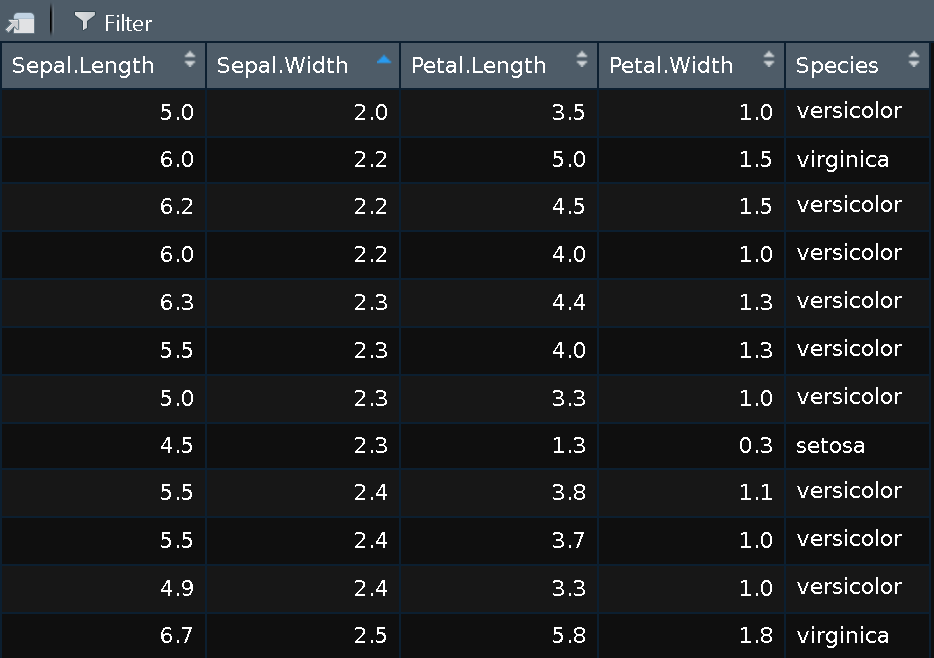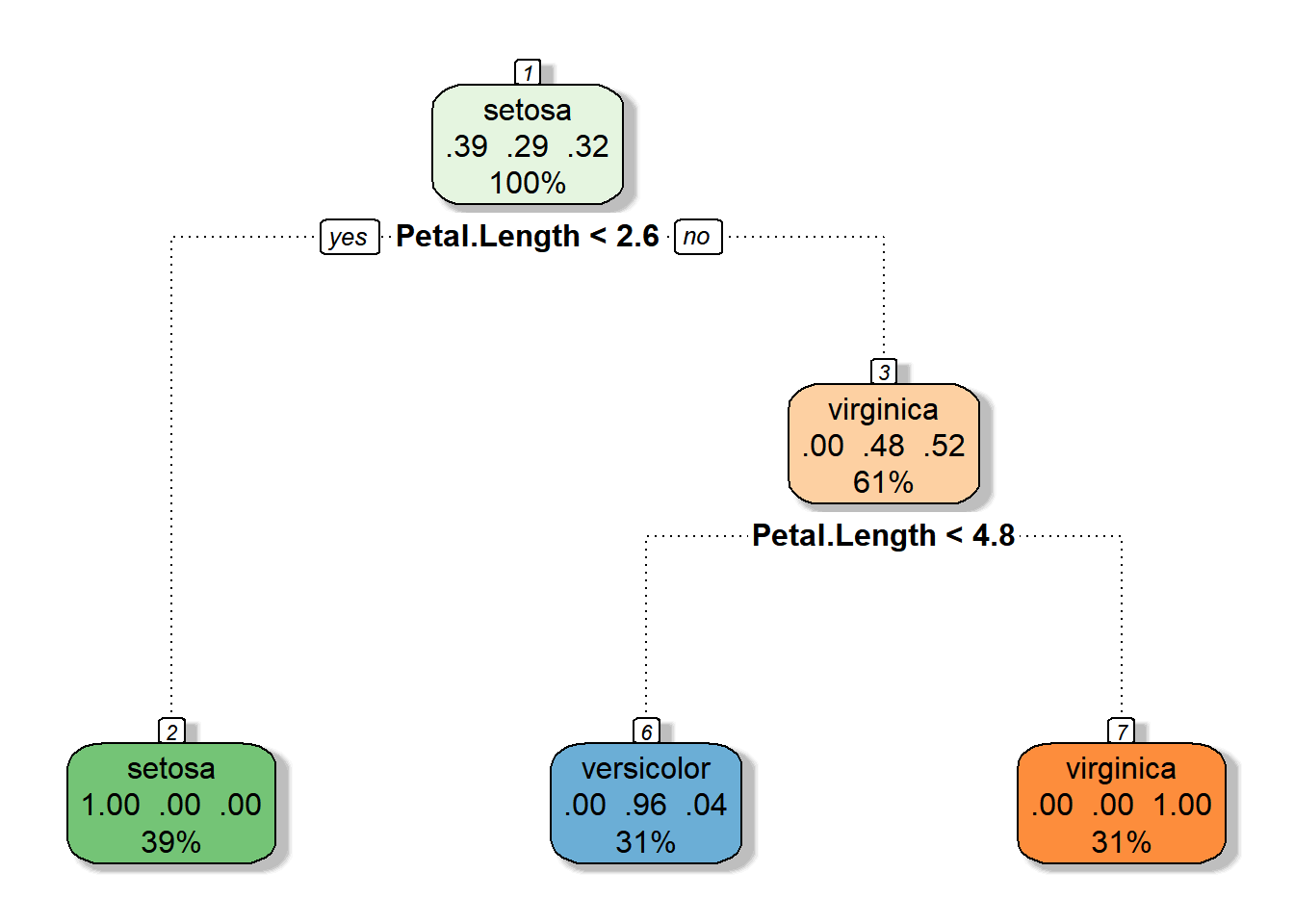#### Videos

Topic Access

StatQuest: Decision Trees

Construct trees with GINI Impurity
for
Ratio interval
Ordinal
Nominal Level Data

No flaws detected

(17  min)

StatQuest: Decision Trees, Part 2 – Feature Selection and Missing Data

(5 min)

Decision Tree 1: how it works (9 min)

assumes that the tree end with pure sets !!!

Entropy discussed in a shallow way

Look at entropy from Thermodynamics point of view: https://www.grc.nasa.gov/www/k-12/airplane/entropy.html

https://en.wikipedia.org/wiki/Entropy_in_thermodynamics_and_information_theory

https://en.wikipedia.org/wiki/Entropy_(information_theory)

https://towardsdatascience.com/entropy-is-a-measure-of-uncertainty-e2c000301c2c

A Gentle Introduction to Information Entropy URL: https://machinelearningmastery.com/what-is-information-entropy/
Decision Tree (CART) – Machine Learning Fun and Easy (9 min) https://www.youtube.com/watch?v=DCZ3tsQIoGU

Decision Tree In R (46 min)

by Simplilearn

last 15 minutes not useful for students

Pruning Classification trees using cv.tree ( 15 min) https://www.youtube.com/watch?v=GOJN9SKl_OE
Machine Learning and Decision Trees ( first 15 minutes ) https://www.youtube.com/watch?v=RmajweUFKvM

#### Articles

Medium Topic Access
Tutorial Classification Trees https://daviddalpiaz.github.io/r4sl/trees.html
Tutorial A Complete Guide on Decision Tree Algorithm https://www.edureka.co/blog/decision-tree-algorithm/
Tutorial Decision Tree: How To Create A Perfect Decision Tree? https://www.edureka.co/blog/decision-trees/
Tutorial What is Overfitting In Machine Learning And How To Avoid It? https://www.edureka.co/blog/overfitting-in-machine-learning/
Tutorial Decision Tree in R with Example https://www.guru99.com/r-decision-trees.html
Tutorial Decision Tree and Random Forests https://www.analyticsvidhya.com/blog/2016/02/complete-tutorial-learn-data-science-scratch/
Tutorial Classification & Regression Trees http://www.di.fc.ul.pt/~jpn/r/tree/tree.html

### rattle::fancyRpartPlot() is good

https://www.gormanalysis.com/blog/magic-behind-constructing-a-decision-tree/

library(rattle)
library(rpart.plot)
library(RColorBrewer)
library(rpart)
mytree <- rpart(
Fraud ~ RearEnd,
data = train,
method = "class"
)
# plot mytree
rattle::fancyRpartPlot(mytree, caption = NULL)
 
library(rpart)

# Load the iris data that we've been working with
data(iris)

# Set the random number seed so that the results
# are the same each time we run the example (if we
# didn't set this the results might differ a bit each time
# because the subsampling process below is stochastic)
set.seed(2568)

Next, we will split our data into training data that we use to fit the model, and test data we use to validate the model. To do this, we will randomly select rows from our dataframe and assign them to the training data. All unsampled rows will be assigned to the test data set.

# Define a variable to subsample rows of the
# iris dataframe
n <- nrow(iris)

# Define a random subsample of the original data.
# We will use these data as a training data set,
# that we will then use to fit the model. The object
# train is just a random sample of row numbers from
# the total number of rows ini the iris data.
# Here, we take half the data
train <- sort(sample(1:n, floor(n / 2)))

# We can define separate data frames for the training
# data and the test data using the indices contained
# in train.

# Training data
iris.train <- iris[train, ]

# Test data
iris.test <- iris[-train, ]

Then, we can fit the classification tree to the training data.# Fit the tree
iris_cart <- rpart(Species ~ ., # Formula: '.' means entire dataframe
data = iris, # Using iris data
subset = train # More specifically, the training data
)



By default, rpart uses gini impurity to select splits when performing classification. (If you’re unfamiliar read this article.) You can use information gain instead by specifying it in the parms parameter.

 mytree <- rpart( Fraud ~ RearEnd, data = train, method = "class", parms = list(split = 'information'), minsplit = 2, minbucket = 1 )

https://www.gormanalysis.com/blog/decision-trees-in-r-using-rpart/


# Install and load the packages library(rattle) # We'll use this one to make some more functional plots library(RColorBrewer) # We'll use this one for colors

# Now, make it fancy
fancyRpartPlot(iris_cart, main = "", sub = "")

in the tree below

the first node include 100% of observations in which setosa species is most frequent

A decision is made based on Petal.Length<2.6

Node 2 has only Setosas 29% of all observations and 100% of it are setosa

Node 3 is composed of two species with a 52% virginica, 61% of all observations are here

A decision is made based on Petal.Length<4.8

Node 6 has .31% of observation in which 96% are versicolor

Node 7 has 31% of observation in which 100% are virginca### also Library(party) is good    https://www.youtube.com/watch?v=GCXsKNMDy1w